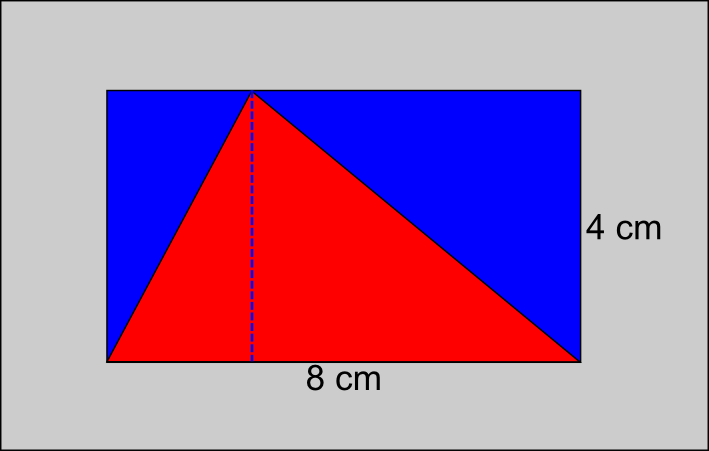The Singing Hedgehog Guide to:
Area - Triangles

The area of any triangle is found by creating a suitable rectangle
and dividing it by two.First we draw a rectangle that just encloses the triangle.
We can see that the red triangle has been split into two smaller
triangles which are the same size as the adjacent blue triangles.
Obviously the area of the triangle is half the area of the rectangle.

To work out the area of a triangle we use a simple formula:

area = base x height ÷ 2

Notice that the height is the perpendicular [at right angles]
distance from the base to the top and not one of the sides.

So for this triangle:area = 8 x 4 ÷ 2 = 16 cm²

next page